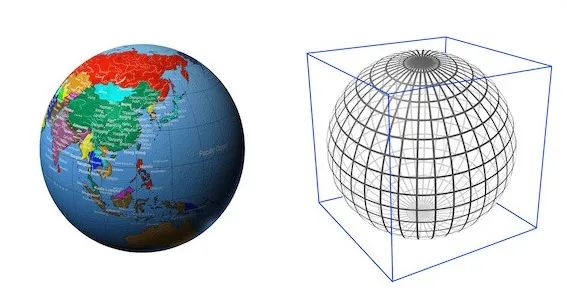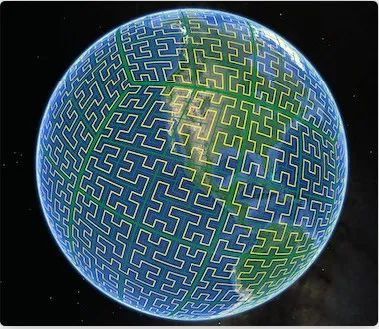# Geospatial Data 在 Nebula Graph 中的实践

TeamD

0收藏

>>>>什么是 Geospatial Data

Nebula Graph 在 2.6 版本中引入了对 Geospatial Data 完整的支持，包括地理空间数据的存储、计算，以及索引。Nebula Graph 目前支持 Geography 类型的地理空间数据，Geography 类型是建模在地球空间坐标系上由经纬度坐标对表示的地理位置信息。

Geospatial Data -- 地理空间数据的使用

Nebula Graph 目前支持点、线、多边形三种空间数据类型。下面介绍一下如何如何创建 Geography 类型属性以及如何插入地理空间数据 Nebula Graph 中。

``````CREATE TAG any_shape(geo geography);
CREATE TAG only_point(geo geography(point));
CREATE TAG only_linestring(geo geography(linestring));
CREATE TAG only_polygon(geo geography(polygon));``````

geography 属性后面没有指定具体的地理形状信息时，代表该列可以存储任意地理形状的数据；当指定形状类型时，则代表只能存储该形状的地理数据，比如 geography (point)，就代表该列只能存储 point 形状的地理位置信息。

``````INSERT VERTEX any_shape(geo) VALUES "101":(ST_GeogFromText("POINT(120.12 30.16)"));
INSERT VERTEX any_shape(geo) VALUES "102":(ST_GeogFromText("LINESTRING(3 8, 4.7 73.23)"));
INSERT VERTEX any_shape(geo) VALUES "103":(ST_GeogFromText("POLYGON((75.3 45.4, 112.5 53.6, 122.7 25.5, 93.9 28.6, 75.3 45.4))"));``````

``INSERT VERTEX only_point(geo) VALUES "201":(ST_Point(120.12，30.16)"));;``

``INSERT VERTEX only_linestring(geo) VALUES "302":(ST_GeogFromText("LINESTRING(3 8, 4.7 73.23)"));``

``INSERT VERTEX only_polygon(geo) VALUES "403":(ST_GeogFromText("POLYGON((75.3 45.4, 112.5 53.6, 122.7 25.5, 93.9 28.6, 75.3 45.4))"));``

``````(root@nebula) [geo]> INSERT VERTEX only_polygon(geo) VALUES "404":(ST_GeogFromText("POINT((75.3 45.4))"));
[ERROR (-1005)]: Wrong value type: ST_GeogFromText("POINT((75.3 45.4))")``````

POINT(120.12 30.16) 代表一个东经 120°12′，北纬 30°16′ 的地理位置点；ST_GeogFromText 函数会从 wkt 参数中解析并构造一个 geography 数据对象，然后 INSERT 语句会将其以 WKB（Well-Known Binary）标准存储在 Nebula Graph 中。

Geospatial functions -- 地理空间函数

Nebula Graph 支持的地理空间函数可以分为以下几大类:
●  构造函数
◆  ST_Point(longitude, latitude)，根据一对经纬度构造一个 geography point 对象
●  解析函数
◆  ST_GeogFromText(wkt_string)，从 wkt 文本中解析 geography 对象
◆  ST_GeogFromWKB(wkb_string)，从 wkb 文本中解析 geography 对象 # 尚未正式支持，因为 Nebula Graph 还未支持二进制字符串
●  格式设置函数
◆  ST_AsText(geogrpahy)，将 geogrpahy 对象以 wkt 文本格式输出
◆  ST_AsBinary(geography)，将 geography 对象以 wkb 文本格式输出 # 尚未正式支持，因为 Nebula Graph还未支持二进制字符串
●  转换函数
◆  ST_Centroid(geography)，计算 geography 对象的重心，重心是一个 geography point 对象
●  谓词函数
◆  ST_Intersects(geography_1, geography_2)，判断两个 geography 对象是否相交
◆  ST_Covers(geography_1, geography_2)，判断第一个 geography 对象是否完全覆盖第二个
◆  ST_CoveredBy(geography_1, geography_2)，ST_Covers 的反义词
◆  ST_DWithin(geography_1, geography_2, distance_in_meters)，判断两个 geography 对象的最短距离是否小于给定距离
●  度量函数
◆  ST_Distance(geography_1, geography_2)，计算两个 geography 对象之间的距离

Geospatial index -- 地理空间索引

Nebula Graph 使用 Google S‍2 库做空间索引。

S2 库将地球表面投影到一个外切的正方体上，然后对正方体的每一个正方形表面递归地进行 n 次四等，最后使用一条空间填充曲线--希尔伯特曲线去连接这些小正方格子的中心。

S2 库使用的是 30 阶的希尔伯特曲线。``CREATE TAG any_shape_geo_index on any_shape(geo)``

``LOOKUP ON any_shape WHERE ST_Intersects(any_shape.geo, ST_GeogFromText("LINESTRING(3 8, 4.7 73.23)"));``

any_shapegeo 列上没有空间索引时，该语句会先将 any_shape 的所有数据读到内存，然后用来计算是否和点(3.0, 8.0)相交，这个计算的开销一般是比较昂贵的。当 any_shape 的数据量较大时，计算开销将难以接受。